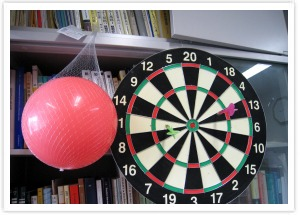# Perspectives in Mathematical Sciences I

Lecturer Hiroyuki OCHIAI, Professor Graduate School of Mathematics, 2009 Spring School of Mathmatics (2・1.5 hours / session One session / week 15 weeks / semester)### Key Features

This course is one of the English lessons conducted by the mathematical division. Personally, I(Ochiai) am not good at English, but because English is essential in mathematical research, I have, to an extent, experience in English mathematical papers, English lectures, and asking questions (answering them, too) in English. By beginning to use English in mathematics at an early stage in the student's academic education, I hope to lessen the effects of 'English allergy', and hopefully lead the students off to a smooth start in mathematical research. If conditions permit, I would like to make the lessons more interactive, with more emphasis on exercises.

### The Purpose of the Course

We denote by SL(2,R) the set of all regular real matrices of size two. SL(2,R) is a group and a non-compact manifold, that is, one of typical examples of non-compact connected simple Lie groups. We survey the classification of equivalence classes of irreducible unitary representations of SL(2,R), which has been established more than 50 years ago.

### The Plan of the Course

This is the first part of three series of lectures. We start from the linear fractional transformation. By differentiating the group action induced on the function spaces, we introduce the simple three-dimensional Lie algebra sl(2) and its representations. The correspondence between Lie groups and Lie algebras are explained in this example. We also compute the basic material on the structure of Lie algebras, such as Killing forms and Casimir operators. We analyze the representations constructed here by using weights and raising/lowering operators. We discuss the irreducibility and the unitarity. As a conclusion, the irreducible unitary representations of SL(2,R) is classified into the following classes: principal series representations (spherical and non-spherical), complementary series representations, (holomorphic and anti-holomorphic) discrete series representations and limit of discrete series representations, and trivial representations.

### Keywords

Lie group, Lie algebra, weight, irreducible, unitary, representation

### Required Knowledge

Level 1 is assumed. [An explanation of Level in Department of Mathematics is given in the web page http://www.math.nagoya-u.ac.jp/. See the precise description in core-2.]

 R. Howe and E.C. Tan, Non-abelian Harmonic Analysis, Springer Verlag.
 A. Knapp, Representation Theory of Semisimple Groups, Princeton University Press.
 is the textbook. A part of  will be discussed in the lecture.
 is the reference book. The full detail of the story is given in .

### Course Schedule

date Contents
4/16 correspondence of Lie algebra within the Lie group
4/23 operation of groups and differential representation
4/30 representations of sl(2) and their weights
5/7 unitary, the definition of irreducibility and their classification

*The remaining sessions are conducted by other instructors.

Session #1

Session #2

Session #3

Session #4